11.3.  New Theory of   low voltage  of Process of  Water Electrolysis

Having the obtained information at our disposal let us begin the search of a new structure of water molecule and a new theory of the process of its electrolysis. This theory should eliminate the existing contradictions in the description of water electrolysis and  give replies to the following fundamental questions:

1 - why do the theoretical calculations demonstrate availability of additional energy during water electrolysis and why do the existing industrial electrolyzers fail to generate it?

2 - why do the existing theoretical values (264-265) of binding energies of hydrogen atoms in water molecules fail to correspond to experimental values of these energies during water electrolysis?

First of all, in order to find replies for these questions it is necessary to have a theory, which would allow to calculate energies of chemical bonds of the electrons with atomic nuclei when they are at any energy level. As the atoms of hydrogen and oxygen play the main role during water electrolysis, we’ll determine binding energies of their electrons with atomic nuclei. We have given some of these calculations, but as they are very important, we’ll give them once more having added a new information.

Taking into consideration that ionization energy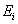of  hydrogen atom is equal to binding energy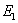of the electron with the nucleus corresponding to the first energy level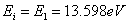and using formulas (212) and (213) we’ll get energies of the photons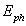emitted or absorbed by the electron, and binding energies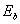of the electron with the atomic nucleus corresponding to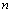energy levels (Table 35).

Table 35. Spectrum of hydrogen atom

 Value n 2 3 4 5 6(exp) eV 10.2 12.09 12.75 13.05 13.22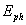(theor.) eV 10.198 12.087 12.748 13.054 13.22(theor.) eV 3.4 1.51 0.85 0.54 0.38

As it is clear (Table 35), there are no energieswhich are obtained by the chemists in their calculations (266-269) in the row of binding energies of the electron with the nucleus of hydrogen atom. But energies similar to experimental value (1.59 eV) by which gases are released during water electrolysis are available in the row of binding energies of the electron of hydrogen atom (1.51 eV) (Table 35) and the eighth electron of oxygen atom (1.53 eV) (Table 36). These energies correspond to the existence of the electrons on the third energy levels.

Thus, among binding energies of the electron of hydrogen atom with its nucleus there are energies  (1.51eV) similar to experimental  value (1.59eV). Let us determine the same energies for the oxygen atom electrons.

As the surface electrons  of the atoms take part in chemical reactions namely, let us consider only the calculation of energies, the absorbed and emitted photons as well as binding energiesof the electrons with the nuclei of the first two surface electrons of oxygen atom.

Ionization energy of the first electron of oxygen atom is equal to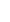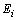=13.618 eV, and its binding energy with the atomic nucleus corresponding to the first energy level is equal to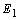=13.752 eV. Energy indices calculation of this electron according to the formulas (212) and (213) gives the following results (Table 36).

Table 36. Spectrum of the 1th electron of oxygen atom

 Value n 2 3 4 5 6(exp) eV 10.18 12.09 12.76 13.07 13.24(theor.) eV 10.16 12.09 12.76 13.07 13.24(theor.) eV 3.44 1.53 0.86 0.55 0.38

As it is clear, binding energies of the first electron of oxygen atom (Table 36) practically coincide with the corresponding binding energies of the electron of hydrogen atom (Table 35). The energy corresponding to the third level (1.53eV) is similar to experimental value of gas release energy (1.59 eV) during water electrolysis. Theoretical values of binding energies of the electron of the first atom of hydrogen and the first electron of oxygen atom in water molecule  obtained on the grounds of spectrum formation law (212) and (213) are similar to the experimental values of this energy.

Now we have every reason to suppose that the first  electron of oxygen atom establishing the bond with the first atom of hydrogen in water molecule is on the third (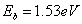) energy level (Table 36).

When we have analysed the regularity of the change of binding energies of the electrons of the oxygen atom and other atoms with their nuclei, we have found that they have almost equal binding energies with the atomic nuclei in case all electrons are present in the atom. That’s why we’ll consider that water molecule symmetry provides equal binding energies with the nucleus of its first electron and the second one.

Thus, we have removed the second contradiction between the theory and the experiment in water electrolysis. Now experimental value of binding energy of the electron of  hydrogen atom with the first electron of oxygen atom in water molecule coincides with theoretical value of this energy.

Low voltage process of water electrolysis takes place when voltage is (1.6-2.3) V and strength of current of hundreds of amperes. Large strength of current proves large expenditure of electrons. As the first electron of the oxygen atom is situated from its nucleus at the longest distance than other electrons, the proton of the hydrogen atom, which is bond with this electron, is the first to come nearer to the cathode and gets the electron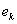from it (Fig. 78, a). After each of two water molecules gets the electron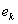, their surface electrons are united at once and form a cluster of two water molecules (Fig. 78, a, b), which are connected by two electronsemitted by the cathode. As it is clear, the orthohydrogen molecule is in the chain of  the protons and the electrons, which unite two water molecules (Fig. 78, a, b). As the electrons, which have arrived from the cathode, have passed the free state phase, hydrogen molecule fusion in this chain is accompanied by release of energy.

It is clear from Fig. 78 that two electronsemitted by the cathode are used for the formation of one hydrogen molecule. In accordance with Faraday’s law, in this case two faraday coulombs of electricity are used for the formation of one mole of hydrogen 2F=2×96485=192980 or 192980/3600=53.60 A×h/mol. If electrolysis takes place at voltage of 1.70 V, for production of one mole of hydrogen E=I×V=53.6×1.70=91.12 W×h will be spent, and for production of 1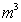E=(1000/22.4)×91.12=1476 kJ/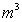=4.10 kWh will be spent.

As it is clear, the calculations with the use of Faraday’s law give a result, which coincides with the experiment almost completely. If hydrogen formation were accompanied by the process of fusion of its molecules, energy would be released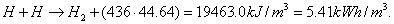If we take into account only the hydrogen molecule fusion and do not take into account the oxygen molecule fusion, heat energy efficacy index should be as follows: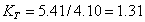.

But it is well known that total heat energy efficacy index of modern electrolysers is less than unit. Why? We’ll try to find an answer to this question (Fig. 78).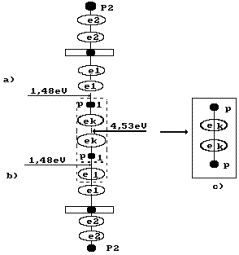Fig. 78. Diagram of formation of the orthogydrogen molecule (s. Fig. 53, a)

Fusion energy of one mole of the hydrogen molecules is equal to 436 kJ. Let us convert this energy in electron volts in the calculation per molecule .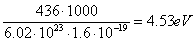.                                            (271)

The value of this energy is shown to the right of the hydrogen molecule situated in a cluster chain (Fig. 78). To the left, binding energies 1.48 eV of the hydrogen atoms with the oxygen atoms in water molecule are shown. Energy 4.53 eV of fusion of the hydrogen molecule redistributes binding energies in the cluster chain in such a way that binding energies 1.48 eV of the hydrogen atoms with the oxygen atoms in water molecules become equal to zero, and the orthohydrigen molecule is separated from the cluster chain (Fig. 78, c).

Thus, the difference between energy 4.53 eV of fusion of the hydrogen molecule and total binding energy (1.48+1.48)=2.96 eV becomes equal to (4.53-2.96)=1.57 eV. This energy is spent to heating the electrolytic solution.

That’s why during 1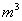of hydrogen is released not 44.64x436=19463 kJ will be released, but the following quantity of energy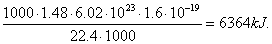(272)

Near the cathode the following chemical reaction will take place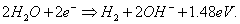(273)

It is  natural that quantity of heat energy 4058 kJ is a part of total energy 14760 kJ, which is spent for production of one cubic metre of  hydrogen . Thermal effectiveness index of this process is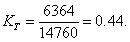(274)

If we take into account that energy content of one gram of hydrogen is equal to 142 kJ and cubic metre of this gas weighs 90 g, total energy effectiveness index will be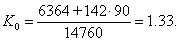(275)

We should note that we have not taken into consideration energy content of oxygen being obtained, because oxygen from the air is usually used when burning hydrogen.

Other variants of hydrogen molecules formation are possible. For example, let us pay attention to the structure of orthohydrogen, which is shown in Fig. 53, b. This structure is formed when the first hydrogen atoms of two water molecules of the first model come nearer to each other (Fig. 72) without the electron emitted by the cathode, i.e. without direct consumption of electric energy for hydrogen molecule formation (Fig. 79). According to Faraday’s law, energy consumption for such hydrogen

molecule formation process is equal to zero.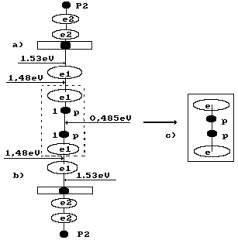Fig. 79. Diagram of formation of the second model  of orthohydrogen  (s. Fig. 53, b); a) and

b) of the diagram of water molecules;  c) orthohydrogen

In this case, each of two water molecules gives one proton and one electron to the hydrogen molecule, and the hydrogen molecule is formed without electrons emitted by the cathode, i.e. without direct expenses of electric power for this process. In this case, electric power is spent only for the separation of the hydrogen molecule being formed from two water molecules. Energy consumption for production of one cubic meter of hydrogen is as follows: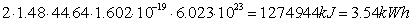(276)

One cubic metre of hydrogen weighs 90 g. Energy content of one gram of hydrogen is equal to 142 kJ. When this hydrogen is burnt, energy of 90×142=12780 kJ will be released. Total index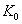of energy efficacy of the process will be as follows: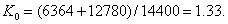(277)

If hydrogen were released as a result of fusion of its molecules, energy would be generated :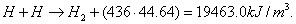(278)

Electrolyzers consume 4 kWh of electric power or (3600x4)=14400 kJ in order to produce one cubic meter of hydrogen. Taking into consideration energy (19463.0) of fusion of one cubic meter of hydrogen and energy (14400) spent for its production, we’ll find  the index of water electrolysis process efficacy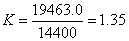.                                                           (279)

Now let us consider how a parahydrogen molecule is formed (Fig. 53, c). Electronemitted by the cathode (Fig. 80) combines two molecules of water. There is a hydrogen molecule structure in the chain being formed. It is formed by the proton of the hydrogen atom of one water molecule, the electronemitted by the cathode and the proton with its electron (the hydrogen atom) of the second water molecule. Thus, one electron emitted by the cathode is spent for the formation of one parahydrogen molecule.

As only one electron is spent for formation of the parahydrogen molecule shown in Fig. 80, according to Farady’s law energy expenses for such process should be half. The calculation according to our method gives an actual result (276).

If the hydrogen molecules are formed according to the diagrams shown in Fig. 78, 79 and 80, there are no hydrogen atom protons and hydrogen atoms  in free state in the solution. There are no processes of fusion of the atoms and the hydrogen atoms, there is no additional energy, which corresponds to these processes. An index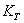of energy efficacy of such processes of water electrolysis will be less than one.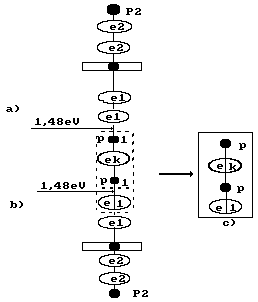Fig. 80. Diagram of parahydrogen molecule formation (s. Fig. 53, c):

and b) water molecules; c) parahydrogen molecule

Let us consider the reactions, which take place near the anode. It is known that hydroxyl ion (Fig. 75) having the negative charge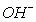moves to the anode (Fig. 81, a). Two hydroxyl ions give one electron each to the anode, connect with each other and form hydrogen peroxide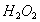(Fig. 81, b).

It is known that the process of formation of hydrogen peroxide is an endothermic one, and the process of formation of oxygen molecule is an exothermic one. When one cubic metre of hydrogen is produced, the process of formation of hydrogen peroxide requires 22.32 x 109.00= 2432.88 kJ. Due to it, even during plasmaelectrolytic process the temperature of the solution in the anode area remains low.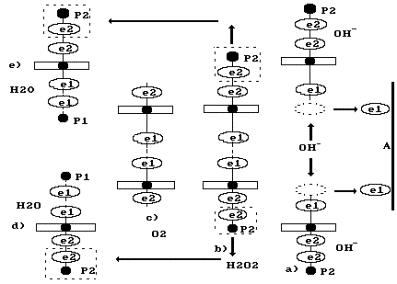Fig. 81. Diagrams: a) transfer of electrons e1 by the ionsto the anode A; b) formation of hydrogen peroxide; c) formation of oxygen molecule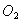and two molecules of water d) and e)

If the oxygen molecule fusion process existed, 22.32x495.00=11048.40 kJ would be released during production of one cubic metre of hydrogen in the anode area. If we subtract energy absorbed during hydrogen peroxide fusion from this value, we’ll get 11048.40-2432.88=8615.52 kJ. If we add this energy to hydrogen molecule fusion energy of 19463.00 kJ, we’ll get 28078.52 kJ. In this case total index of energy efficacy should be as follows: 28078.52/14400=1.95. As this energy does not exist in reality, this fact confirms a hypothesis concerning the lack of the hydrogen molecule fusion process in the cathode area and the oxygen molecules in the anode area. The hydrogen molecule (Figs 78, 79 and 80) and the oxygen molecule (Fig. 81, b) are formed prior to a release into the free state, that’s why energy of their fusion is not generated.

When two electrons are transferred to the anode by two ions of hydroxyl (Fig. 81, a), a hydrogen peroxide molecule is formed (Fig. 81, b), which decomposes and forms an oxygen molecule (Fig. 81, c) and two hydrogen atoms; they are combined with the hydroxyl ions and form two water molecules (Fig. 81 d, e) If we take it into account, the chemical reaction in the anode area will be written in such a way: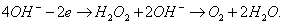(280)

Thus, we have removed the contradictions of the existing theory of low-voltage process of water electrolysis and have worked out a new theory, which describes this process in detail and reflects reality more accurately.

The Foundations of Physchemistry of Microworld

Ó2003 Kanarev Ph. M.

Internet Version - http://book.physchemistry.innoplaza.net

<< Back to Physchemistry Book Index